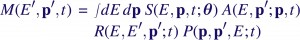#### Equation of the Week: Confronting data with model

Starting a new feature — highlighting some equation that is widely used in astrophysics or astrostatistics. Today’s featured equation: what instruments do to incident photons.

Almost all of astrophysics is about inferring some characteristic of the source from the light gathered by some instrument. And the instruments usually give us information on 3 quantities of interest: when the photon arrived, where did it land, and what was its color. In X-ray astronomy in particular, these are usually recorded as a lengthy table of “events”, where each row lists (t, p, E), where t are the arrival times of the photons (which can be measured with a precision anywhere from microseconds to seconds), p=(x,y) says where on which pixel of the detector the photon landed, and E represents how energetic the photon was. Generally, both p and E are blurred, the former because of the intrinsic limitations of how well the telescope can focus and how well the detector can localize, and the latter because of limitations in evaluating the total energy deposited in the detector by the incoming photon. So, in general, the expected signal at the detector can be written as follows:where E is the energy of the incoming photon and E’ is what it is registered as, p is the true sky location and p’ is the registered location, S(E,p,t;theta) is the astrophysical signal, A(E,p’) is the effective area of the telescope/detector combination, P(p,p’;E,t) is the so-called Point Spread Function, and R(E,E’;p) is the energy response matrix.

The expected signal, M(E’,p’,t) is then compared with the collected data to infer the values and uncertainty in theta, the parameters that define the source model. The task of calibration scientists is to compute A, R, and P accurately enough that the residual systematic errors are smaller than the typical statistical error in the determination of the theta.

In general, the calibration products also vary with time, but usually these variations are negligible over the duration of an observation, so you can leave out the t from them. Not always though!